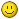## Reply: PM study coach - TCPI demystified

 Name E-mail Your e-mail address will never be displayed on the site. Subject Message [quote="Gerald Derflinger" post=1352]Hi Cornelius, I have a question on the TCPI demystified paper from the PMstudy coach cost mgmt. lesson. On page 3 there is a calculation of the required EAC to be able to calculate the TCPI (based on EAC). Here are the numbers from Frank's dog house: status on day 3: BAC=500 USD EV=300 USD AC=400 USD To calculate TCPI, we first need to calculate EAC. Per definition the EAC=AC+ETC (one possibility). So, corresponding to this example we have to make a new forecast on ETC as we need to earn 2 USD value for every 1 USD we spend (as posted in the example). Therefore new ETC = 400 USD ( 200 USD / day). Now, in the calculation of the EAC there is the EV used instead of AC - so, can you tell me please what is the reason for this? I thought we need AC to calculate the EAC? I look forward to your reply. thanks, Gerald[/quote]

## Topic History of : PM study coach - TCPI demystified

Max. showing the last 6 posts - (Last post first)
9 years 6 months ago #1501

Gerald DerflingerI almost forgot on my entry heresorry for that - comming back to it, maybe does anyone know the answer on it? Or can anyone correct me? (if my thoughts are wrong)

would be really appreciated...

thx,gerald
9 years 8 months ago #1354

Gerald DerflingerThx for feedback first of all.

I agree to your formula in principle, but when you look at the example following to your posted formula, it is necessary also to calculate the TCPI based on EAC:

TCPI = (BAC-EV) / (EAC-AC)

To be able to calculate this formula now, it is necessary to calculate actual EAC first. Therefore 2 possible way would work:

1) EAC = AC + BAC - EV
= 400 + 500 - 300
= 600

2) EAC = AC + ETC
= 400 + 400
= 800

In this example you have 400 USD, 200 USD per day, 2 days left. So, described on the paper it is calculated as following:

EAC = 700 -> (EV + 400) , EV = 300 (3 days are already over, 100 USD per day)

As you can see here, in the example ones is using EAC = EV + ETC instead of EAC = AC + ETC

Do you know why this has been chosen?

thx, gerald
9 years 8 months ago #1353

Nitin ShekharTCPI demontrated overall performance and is calculated by dividing the Total work remaining by Total fund remaining. In this case,
the total work remaining will be BAC-EV and
Total fund remaining will be BAC-AC
thus, the formula is TCPI = (BAC-EV)/(BAC-AC)
9 years 8 months ago #1352

Gerald DerflingerHi Cornelius,

I have a question on the TCPI demystified paper from the PMstudy coach cost mgmt. lesson. On page 3 there is a calculation of the required EAC to be able to calculate the TCPI (based on EAC). Here are the numbers from Frank's dog house:

status on day 3:

BAC=500 USD
EV=300 USD
AC=400 USD

To calculate TCPI, we first need to calculate EAC. Per definition the EAC=AC+ETC (one possibility). So, corresponding to this example we have to make a new forecast on ETC as we need to earn 2 USD value for every 1 USD we spend (as posted in the example). Therefore new ETC = 400 USD ( 200 USD / day).

Now, in the calculation of the EAC there is the EV used instead of AC - so, can you tell me please what is the reason for this? I thought we need AC to calculate the EAC?